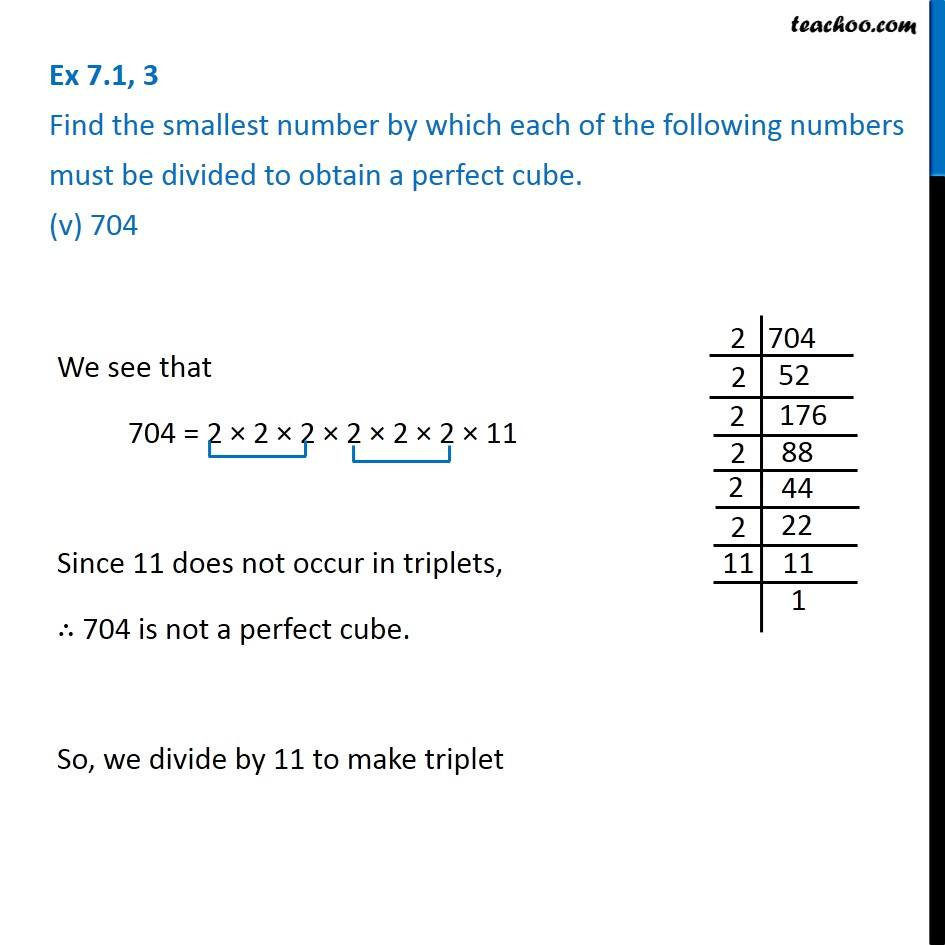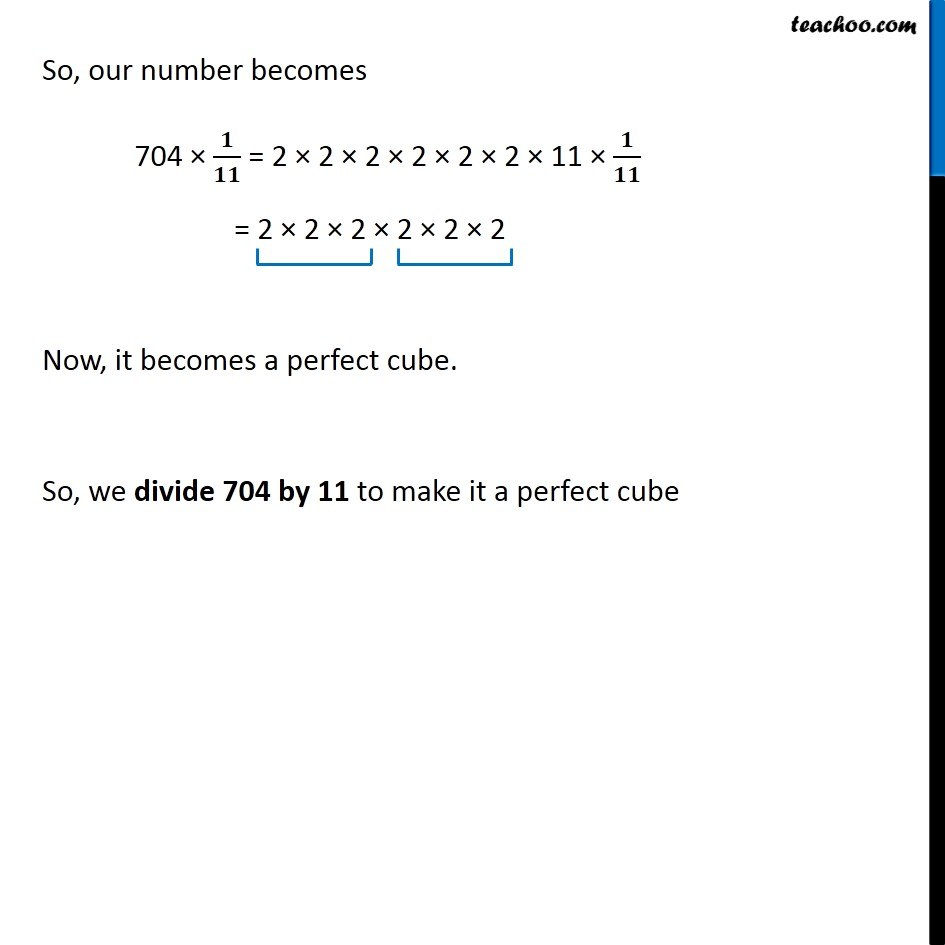Ex 7.1

Chapter 7 Class 8 Cubes and Cube Roots
Serial order wiseGet live Maths 1-on-1 Classs - Class 6 to 12

### Transcript

Ex 7.1, 3 Find the smallest number by which each of the following numbers must be divided to obtain a perfect cube. (v) 704 We see that 704 = 2 × 2 × 2 × 2 × 2 × 2 × 11 Since 11 does not occur in triplets, ∴ 704 is not a perfect cube. So, we divide by 11 to make triplet So, our number becomes 704 × 𝟏/𝟏𝟏 = 2 × 2 × 2 × 2 × 2 × 2 × 11 × 𝟏/𝟏𝟏 = 2 × 2 × 2 × 2 × 2 × 2 Now, it becomes a perfect cube. So, we divide 704 by 11 to make it a perfect cube